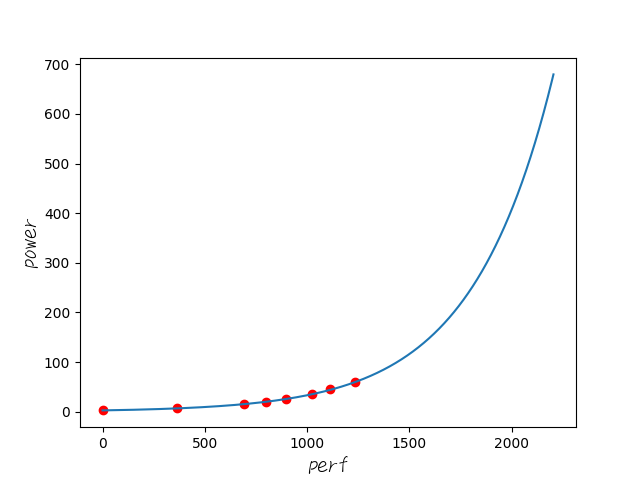# 测试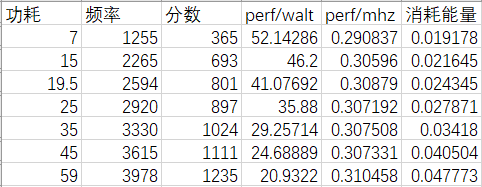# 数据拟合

``````class Net(nn.Module):
def __init__(self):
super().__init__()
self.k = Parameter(torch.tensor(0.0))
self.n = Parameter(torch.tensor(0.0))
self.b = Parameter(torch.tensor(0.0))

def forward(self, x):
return self.k * (self.n ** x) + self.b``````

## 坑一 底数拟合不动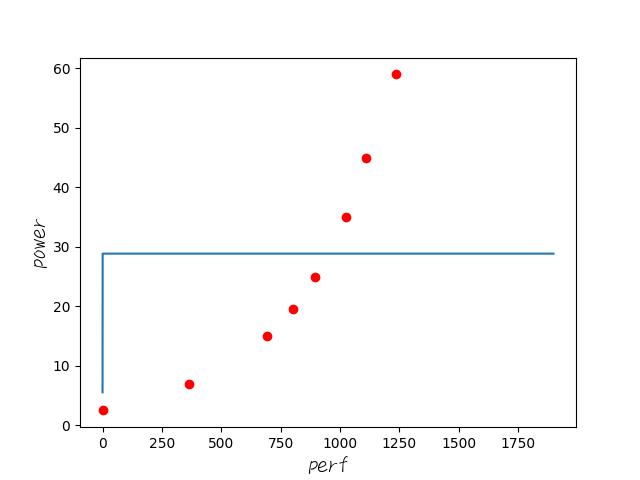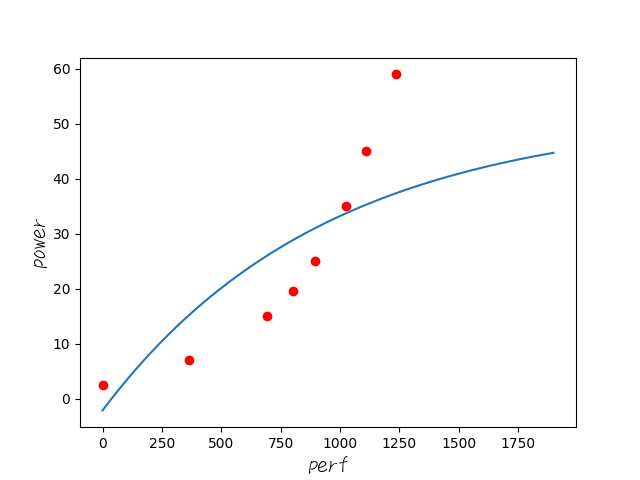## 坑二 损失都显示不出来了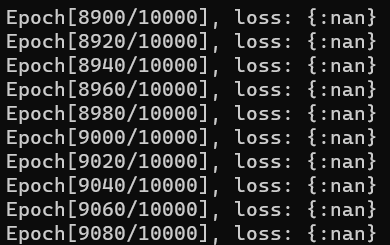`(self.k * (self.n ** (x / 300)) + self.b) * 10`

## 坑三 学习率的选择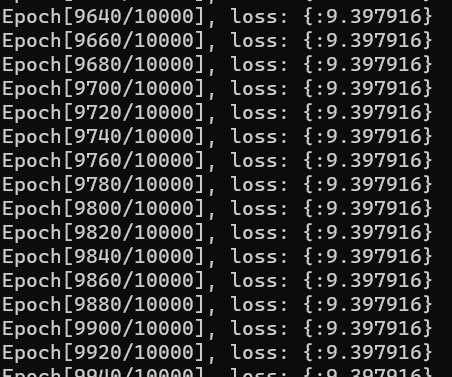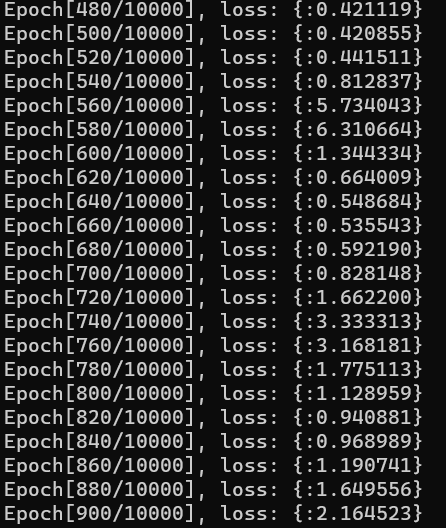## 完成了！

``````#!python

import torch
from torch import nn, optim
import numpy as np
import matplotlib.pyplot as plt
from torch.nn.parameter import Parameter
from matplotlib.font_manager import FontProperties

x_train = torch.tensor([0.0, 365.0, 693.0, 801.0, 897.0, 1024.0, 1111.0, 1235.0])

y_train = torch.tensor([2.5, 7.0, 15.0, 19.5, 25.0, 35.0, 45.0, 59.0])

class Net(nn.Module):
def __init__(self):
super().__init__()
self.k = Parameter(torch.tensor(0.0))
self.n = Parameter(torch.tensor(1.5))
self.b = Parameter(torch.tensor(0.0))

def forward(self, x):
return (self.k * (self.n ** (x / 300)) + self.b) * 10

net = Net()
criterion = nn.MSELoss()
optimizer = optim.SGD(net.parameters(), lr=5 * 1e-6)

num_epochs = 200000

inputs = Variable(x_train)
target = Variable(y_train)
for epoch in range(num_epochs):
out = net(inputs)
loss = criterion(out, target)
loss.backward()
optimizer.step()

if (epoch+1) % 20 == 0:
print("Epoch[%d/%d], loss: {:%.6f}"%(epoch+1, num_epochs, loss.data))

net.eval()
torch.save(net, "net.pkl")
font = FontProperties(fname=r"../math/ITXEWriting.ttf", size=15)
plt.xlabel("perf", fontproperties=font)
plt.ylabel("power", fontproperties=font)
plt.plot(x_train.numpy(), y_train.numpy(), 'ro')
x2 = np.arange(0, 1900, 0.1)
predict = net(torch.from_numpy(x2))
predict = predict.data.numpy()
plt.plot(x2, predict)
plt.show()``````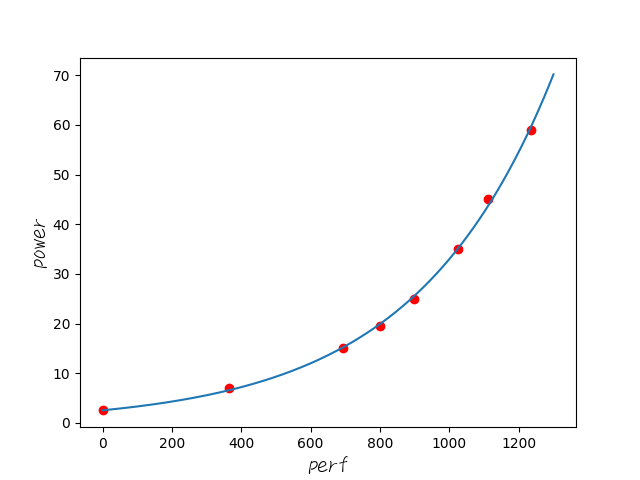loss也在可接受的范围内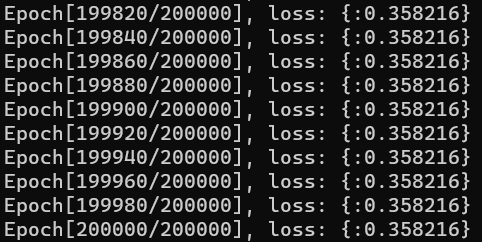# 科技发展大赏

10代U + Zen2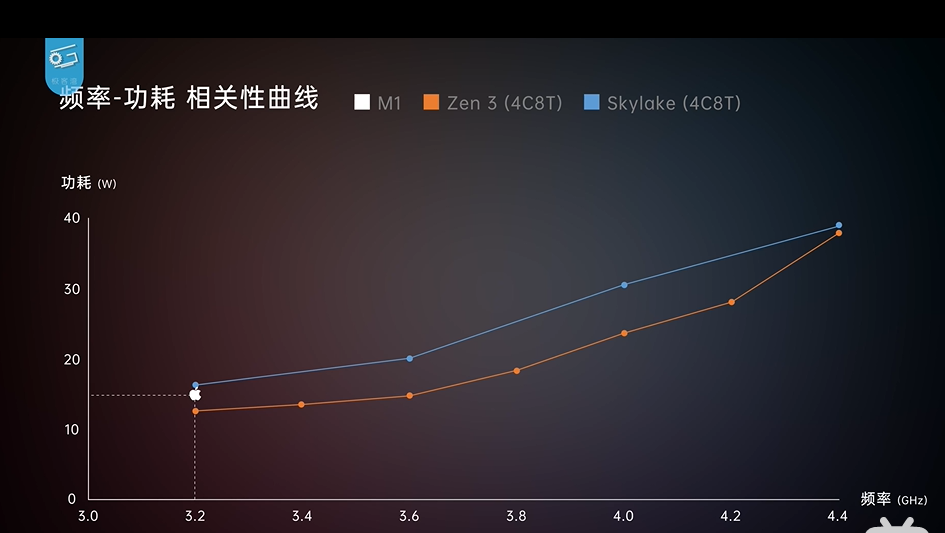（当然，我这是6C12T的，不过换算过去依然是辣鸡）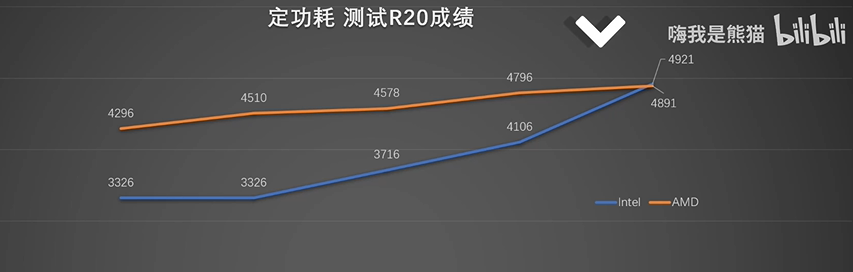（注：9750h的R20成绩在2650左右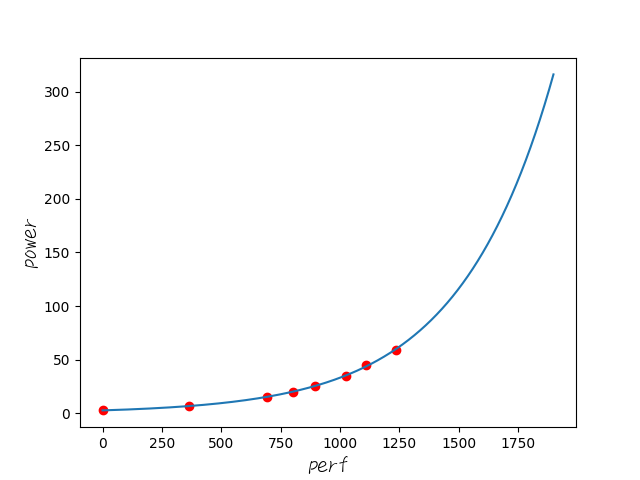300w😅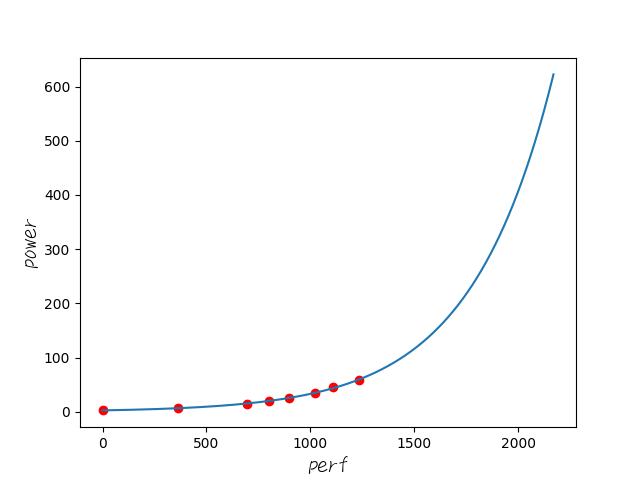600w草 这就是传说中的指数爆炸么。。